# Exponential topology

The weakest topology on the set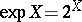of all closed subsets of a topological spacein which the sets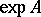are open (in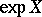) ifis open, and closed (in) ifis closed. If, thendenotes the set of all subsets ofthat are closed in.
Example. The topology of the metric space of closed bounded subsets of a metric space endowed with the Hausdorff metric. The general definition is: Let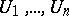be an arbitrary finite collection of non-empty open sets in; a basis for the exponential topology consists of sets of the formwheredenotes the point ofcorresponding to a given closed set. The spaceendowed with the exponential topology is called the exponent of the space. Ifis a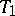-space, then so is. Ifis regular, thenis a Hausdorff space. Ifis normal, thenis completely regular. For the exponential topology normality is equivalent to compactness. If the spaceis compact, then so is. Ifis a dyadic compactum and the weight ofdoes not exceed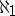, thenis also a dyadic compactum. On the other hand, the exponent of any compactum of weight greater than or equal to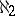is not a dyadic compactum. The exponent of a Peano continuum is an absolute retract in the class of metric compacta and, consequently, it is a continuous image of an interval. However, an exponent of uncountable weight is not a continuous image of the Tikhonov cube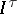. Letbe a closed mapping of a spaceonto a space. The mappingdefined byis called the exponential mapping. Ifis a continuous mapping of a compactumonto a compactum, then it is open if and only if the mapping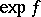is open. The functoracting from the category of compacta and continuous mappings into the same category is a covariant functor of exponential type. Here to a morphismthere corresponds its exponent.